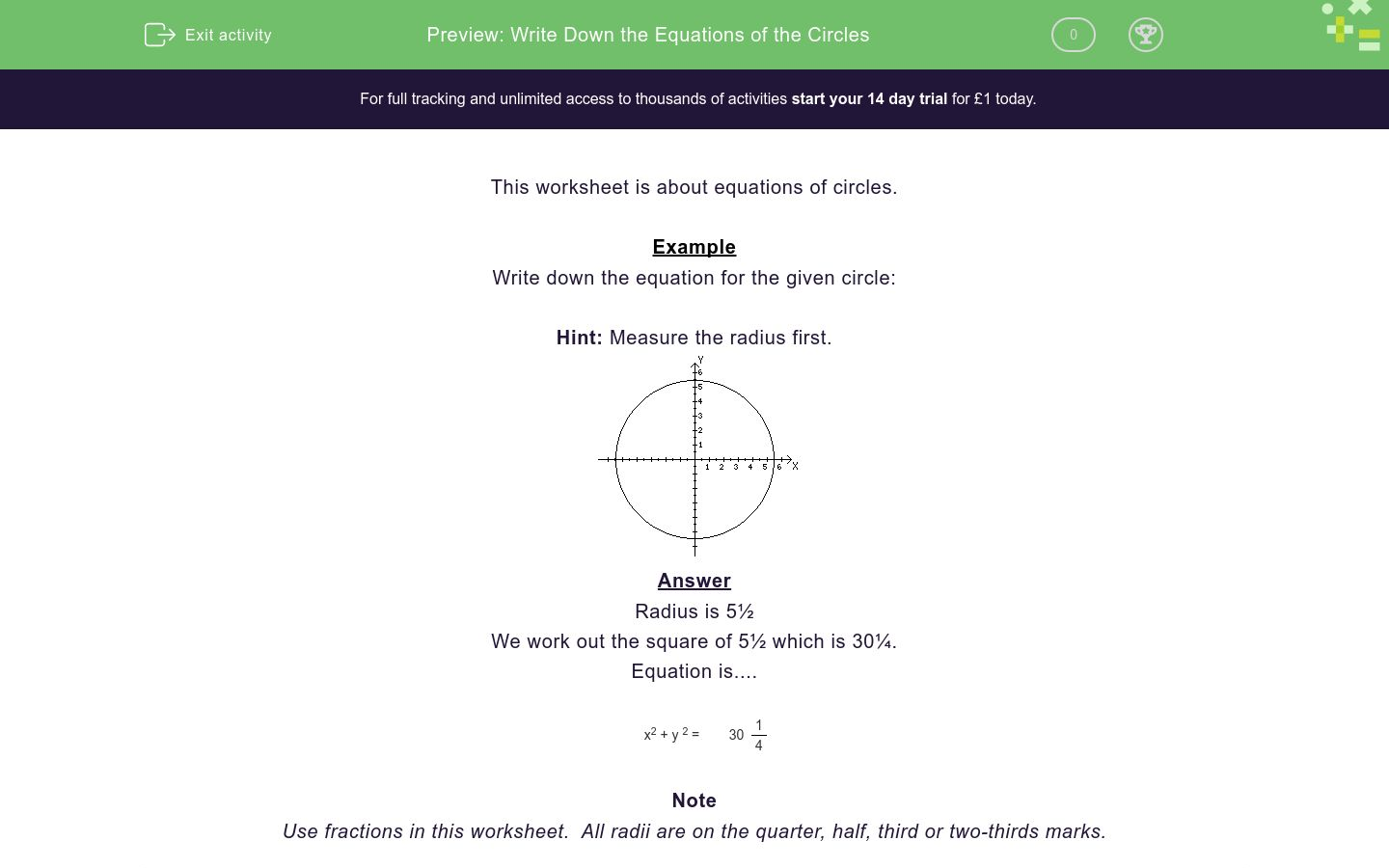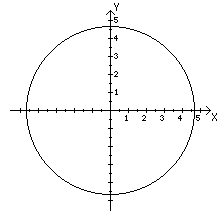# Write Down the Equations of the Circles

In this worksheet, students write down the equations of the given circles.Key stage:  KS 4

Curriculum topic:  Algebra

Curriculum subtopic:  Plot and Interpret Graphs to Resolve Simple Kinematic Problems

Difficulty level:### QUESTION 1 of 10

This worksheet is about equations of circles.

Example

Write down the equation for the given circle:

Hint: Measure the radius first.We work out the square of 5½ which is 30¼.

Equation is....

 x2 + y 2 = 30 1 4

Note

Use fractions in this worksheet.  All radii are on the quarter, half, third or two-thirds marks.

Write down the equation for the given circle:

Hint: Measure the radius first.Write down the equation for the given circle:

Hint: Measure the radius first.Write down the equation for the given circle:

Hint: Measure the radius first.Write down the equation for the given circle:

Hint: Measure the radius first.Write down the equation for the given circle:

Hint: Measure the radius first.Write down the equation for the given circle:

Hint: Measure the radius first.Write down the equation for the given circle:

Hint: Measure the radius first.Write down the equation for the given circle:

Hint: Measure the radius first.Write down the equation for the given circle:

Hint: Measure the radius first.Write down the equation for the given circle:

Hint: Measure the radius first.• Question 1

Write down the equation for the given circle:

Hint: Measure the radius first.EDDIE SAYS
 x2 + y 2 = 28 4 9
• Question 2

Write down the equation for the given circle:

Hint: Measure the radius first.EDDIE SAYS
 x2 + y 2 = 30 1 4
• Question 3

Write down the equation for the given circle:

Hint: Measure the radius first.EDDIE SAYS
 x2 + y 2 = 13 4 9
• Question 4

Write down the equation for the given circle:

Hint: Measure the radius first.EDDIE SAYS
 x2 + y 2 = 18 7 9
• Question 5

Write down the equation for the given circle:

Hint: Measure the radius first.EDDIE SAYS
 x2 + y 2 = 21 7 9
• Question 6

Write down the equation for the given circle:

Hint: Measure the radius first.EDDIE SAYS
 x2 + y 2 = 6 1 4
• Question 7

Write down the equation for the given circle:

Hint: Measure the radius first.EDDIE SAYS
 x2 + y 2 = 12 1 4
• Question 8

Write down the equation for the given circle:

Hint: Measure the radius first.EDDIE SAYS
 x2 + y 2 = 11 1 9
• Question 9

Write down the equation for the given circle:

Hint: Measure the radius first.EDDIE SAYS
 x2 + y 2 = 5 4 9
• Question 10

Write down the equation for the given circle:

Hint: Measure the radius first.